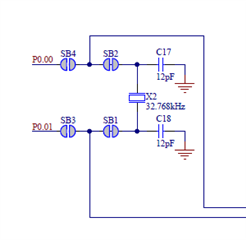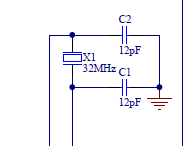# load capacitor calculation of nRF52840 xtals

Hi, there,

Each load capacitors of nRF52840-DK are like below.And i checked BOM file to find out load capacitance of each xtals.

XTAL SMD 2016, 32MHz, Cl=8pF, Tol: ±10ppm, Stab: ±15ppm

XTAL SMD 2012, 32.768kHz, 9pF, ±20ppm

As you know, load capacitor equation is like below.

C1 = C2 = C_L * 2 - (Input capacitance XC1 and XC2 in case of HFXO)

C17 = C18 = C_L * 2 - (Input capacitance on XL1 and XL2 pads in case of LFXO)

So, if i calculate these values based on document,

Input capacitor of HFXO C1=C2=8*2-3pF=13pF

input capacitor of LFXO C17=C18=9*2-4pF=14pF

Input capacitors of nRF52840-DK and values of above are different.

Could anybody answer why this is different?

Thank you.

Related